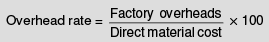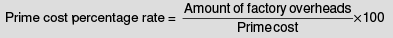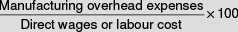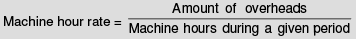Courses

# Methods of Absorbing Production Overheads (Part - 1) - Cost Accounting B Com Notes | EduRev

## Cost Accounting

Created by: Arshit Thakur

## B Com : Methods of Absorbing Production Overheads (Part - 1) - Cost Accounting B Com Notes | EduRev

The document Methods of Absorbing Production Overheads (Part - 1) - Cost Accounting B Com Notes | EduRev is a part of the B Com Course Cost Accounting.
All you need of B Com at this link: B Com

Before we describe the various methods, it would be better to know how to judge whether a method will give good results or not. The method selected for charging overheads to jobs or products should be such as will ensure:

(i) that the total amount charged (or recovered) in a period does not differ materially from the actual expenses incurred in that period. In other words, there should not be any material over or underrecovery of overheads; and

(ii) that the amount charged to individual jobs or products is equitable. In case of factory overheads, this means—

(a) that the time spent on completion of each job should be taken into consideration;

(b) that a distinction should be made between jobs done by skilled workers and those done by unskilled workers. Usually, the latter class of workers need more supervision, as they cause greater wear and tear of machines and tools and waste a larger quantity of materials. Hence jobs done by such workers should bear a correspondingly higher burden for overheads; and

(c) that jobs done by manual labour and those done by machines should be distinguished. It stands to reasons that no machine expenses should be charged to jobs done by manual labour.

In addition, the method should :

(i) be capable of being used conveniently; and

(ii) yield uniform results from period to period as far as possible any change that is apparent should reflect a change in the underlying situation, such as substitution of human labour by machines.

Several methods are commonly employed for computing the appropriate overhead rate to be employed. The common methods are as under:

(1) Percentage of direct materials cost.

(2) Percentage of prime cost.

(3) Percentage of direct labour cost.

(4) Direct labour hour rate.

(5) Machine hour rate.

(6) Combined machine hour and labour hour rate.

(7) Rate per unit of production.

Percentage of direct material cost

In this method the cost of direct materials used in the manufacture of a product is used as the base in absorption of factory overheads. The overhead rate is calculated on the basis of the following formula:This method gives satisfactory results in the following circumstances:

(i) Where the proportion of overheads to the total cost is significant.

(ii) Where the prices of materials are stable.

(iii) Where the output is uniform i.e. only one kind of article is produced.

(i) The calculation of overhead rate is simple as the cost of direct material is easily available.

(ii) This method is more suitable when prices of materials are fairly stable.

(iii) Overhead cost pertaining to upkeep and handling of materials can be absorbed equitably by this method

Percentage on prime cost

An actual or pre-determined rate of overhead absorption is calculated by dividing the overheads to be absorbed by the prime cost incurred or expected to be incurred and expressing the result as a percentage. This is calculated as follows:This method has the advantage of simplicity and is applied because it considers both material and labour which gives rise to overhead expenses.

These two methods are generally considered to be unsuitable on account of the following reasons.

(1) Manufacturing overhead expenses are firstly a function of time, i.e., time is the determining factor for the incurrence and application of manufacturing overhead expenses. The overhead expenses, specially manufacturing expenses, can in the ultimate analysis be regarded as expenditure incurred in providing the necessary facilities and services to workers employed in the productive processes. The question of facilities and services made available to workers naturally is dependent on the length of the time during which the workers make use of these facilities. It may, therefore, be said that the job or product on which more time has been spent would entail larger manufacturing expenses than the job requiring lesser time. This factor is altogether ignored by the first method.

(2) When the overhead cost is allocated as a percentage of direct materials or prime cost, the same is the determining factor. As a result, when there are two jobs, otherwise absolutely similar and requiring same operational time but using materials having varying prices, their manufacturing overhead cost would be different; these should not normally vary if time taken is the same.   The method of apportioning overhead costs on the basis of prime cost also does not take into consideration the time factor. The fact that the amount includes labour cost over and above materials cost, does not render the prime cost any more suitable; in fact, the results are liable to be more misleading because of the cumulative error of using both the labour and materials cost as the basis of allocation of overhead expenses, on neither of which they are dependent.

(3) There is no close or direct connection between the manufacturing expenses and the direct materials cost or prime cost of jobs produced.

(4) Since material prices are prone to frequent and wide fluctuations, the amount of manufacturing overheads recovered, if based on material cost or prime cost, also would fluctuate violently from job to job and from period to period.

(5) The skill of the workers involved and whether machines were used or not, are ignored.

Percentage of direct labour cost

According to this method, the manufacturing overhead expenses are charged as a percentage of the direct wages incurred on jobs. The formula for computing the percentage rate for a period is as follows:The numerator for overhead expenses and the denominator for direct wages may be either an estimated sum, actual amount or normal amount. As has been stated earlier, overhead rates are usually predetermined and the use of actual figures is not very common.

This method also fails to give due recognition to the element of time which is of prime importance in the accounting for and treatment of manufacturing overhead expenses except in so far as the amount of wages is a product of the rate factor multiplied by the time factor. Thus, the time factor is taken to consideration only indirectly or partially in the computation of the overhead percentage rate. This method, therefore, cannot be depended upon to produce very accurate results where the same type of work is performed at the same time by different type of workers, skilled and unskilled, with varying rates of pay. Also no distinction is made between jobs done by manual labour and those done by machines.

Inspite of the inaccuracies which may arise under this method, it is widely used in actual practice, because it is simple and does not involve much calculations; for in costing any job, the labour cost has to be ascertained anyhow. If, on the other hand, a more scientific method is employed, e.g., the labour hour or the machine hour rate, which gives proper allowance to the time element, these would introduce more complexities in the overhead accounting procedure. Thus, the advantage of elimination of a small error in practice may be a heavy price to pay on account of introduction of complexities aforementioned. Also, under this method, there is no large over or under recovery of overheads.

Advantages of Percentage of Direct Labour Cost

(i) The method is simple and economical to apply;

(ii) The time factor is given fair recognition;

(iii) Total expenses recovered will not differ much from the estimated figure since total wages paid are not likely to fluctuate much.

Disadvantages of Percentage of Direct Labour Cost

(i) It gives rise to certain inaccuracies as the time factor is not being given adequate importance;

(ii) Where machinery is used to some extent in the process of manufacture, an allowance for such a factor is not made; and

(iii) It does not provide for varying skills of workers. It is possible to consider the time factor fully by ascertaining the factory overheads per productive labour hour. Suppose the total of direct productive labour hours is 1,50,000 and the factory overheads total `3,00,000, then the productive labour hour rate is Rs 2.

Direct labour hour rate

This method is a distinct improvement on the percentage of direct wages basis, as it fully recognises the significance of the element of time in the incurring and application of manufacturing overhead expenses. This method is admirably suited to operations which do not involve any large use of machinery. A direct labour hour rate is calculated for each category of workers. The expenses incurred, other than wages paid to workers, on each category of workers are listed and totalled for a period. The figure is divided by the number of hours to be put in by that category of workers. Thus, full attention will be paid to the skill of the workers for charging overheads. Productive labour hour rate is a variation of this method. It is computed by dividing the total factory expenses for a period by the total number of hours put in by all the direct workers during that period. Thus, this method, though making no allowance for the skill of workers, gives full recognition to the time factor.

Machine hour rate

By the machine hour rate method, manufacturing overhead expenses are charged to production on the basis of a number of hours a machine or machines are used on jobs or work orders. There is a basic similarity between the machine hour and the direct labour hour rate methods, in so far as both are based on the time factor. The choice of one or the other method is conditioned by the actual circumstances of the individual case. In respect of departments or operations, in which machines predominate and the operators perform relatively a passive part, the machine hour rate is more appropriate. This is generally the case for operations or processes performed by costly machines, which are automatic or semiautomatic and where operators are needed merely for feeding and tending them rather than for regulating the quality or quantity of their output. In such cases, the machine hour rate method alone can be depended on to correctly apportion the manufacturing overhead expenses to different items of production. What is needed for computing the machine hour rate is to divide overhead expenses for a specific machine or group of machines for a period by the operating hours of the machine or the group of machines for the period. It is calculated as follows:Usually, the computation is made on the basis of the estimated expense or the normal expense for the coming period. Thus, the machine hour rate usually is a predetermined rate. Rate for each individual machine may be worked out or, where a number of similar machines are working in a group, there may be a single rate for the whole group.

## Cost Accounting

137 videos|139 docs

,

,

,

,

,

,

,

,

,

,

,

,

,

,

,

,

,

,

,

,

,

;